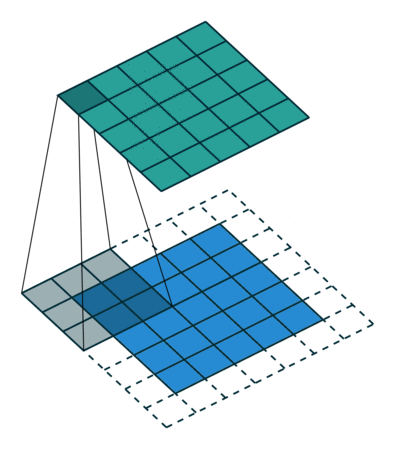# Convolutional Neural Network Basics

14 Oct 2018 - 3 minute read

## Convolution

It is a matrix operation in which we add each element with local neighbors with respect to the weight of the kernels. Mathematically, it is the element-wise product of each element of the kernel with the image-piece followed by a sum. These filters can be used to detect various things like edges etc.

## Filters/Kernels

Filters/Kernels capture features in their receptive field using matrices containing values (weights) with convolution. A higher result of this operation implies that the feature captured by the kernel is in the image, and a lower score implies the opposite.

## Epochs

A single epoch is a combination of one forward pass and a one backward pass through the network and the whole dataset has been covered. A single epoch is usually not enough and leads to underfitting. Too many epochs, would lead to a longer training time and also overfit to the data.

## 1×1 Convolutions

1×1 Convolutions are used to change the dimensionality of the the previous layer. It will take in x channels and not look at any neighbors and take the sum and change it into y channels. This is very useful, and for example, can be used after max pooling to reduce the z-dimensionality.

## 3×3 Convolution3×3 Convolutions are composed of 9 parameters. We tend to use them because they can be combined to have the same effect as higher area convolutions. Back to back application of 3×3 convolution would have the same effective receptive field as would a single 5×5 convolution and would also be faster as this is what has been optimized by modern day GPUs and TPUs.

## Feature Maps

Feature maps, also called activation maps, are the final output results of the activation function (such as ReLU or Sigmoid) applied on the values of a given filter or kernel. A higher activation corresponds to higher likelihood that a feature was found by the kernel in the image and a lower would imply it not being in the receptive field.

## Feature Engineering

In older concepts, people used to manually extract local features also called interest points. This used to utilize various algorithms which used to extract points in the image and would then be used to match similar ones in other images. For example,

The above image shows ORB interest points and then matches these points for another image taken from another angle.

## Activation Function

The purpose of the activation function is to find a function that can take the input signal and squish them between $-1$ and $1$, and get an output signal.

### Hyperbolic Tan function$f(x) = \frac{1-exp(-2x)}{1+exp(-2x)}$

### Sigmoid$f(x) = \frac{1}{1 + exp(-x)}$

### Rectified Linear Units$R(x) = max(0,x)$

There are other modifications of ReLU like Leaky ReLU and randomized leaky ReLU.

## Receptive Fields

Receptive Field of a kernel is the region of the image which affects its result. For layers directly connected to the image, this may be a very small region of 3×3 but for higher layers this region increases as they combine multiple receptive fields below them.